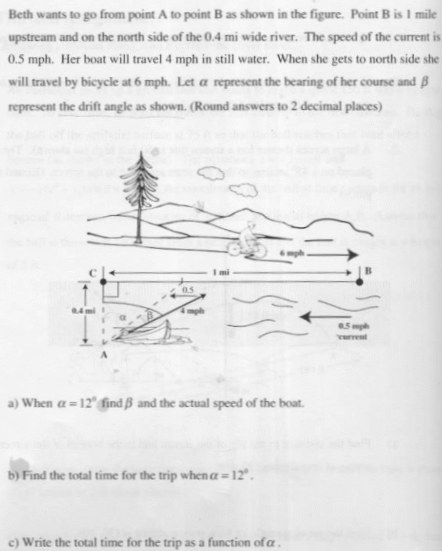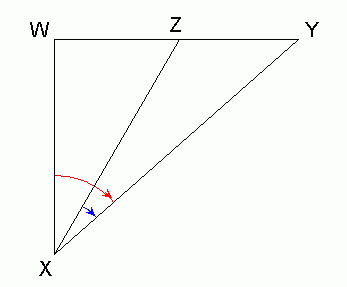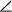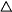SEARCH HOMEMath Central Quandaries & QueriesQuestion from Kimi:I have been working on the attached math problem for my college trig class for over a week. Every avenue I've tried seems to lead either to a dead end, an unreasonable answer, or extremely complicated computations. I was able to calculate the speed of the boat to 3.51 mph, but cannot figure out the measure of angle beta. Once I can figure that out, I can do the rest of the problem. Thanks.Hi Kimi.

It took me a moment to realize that the angle α is all the way across both smaller angles. I've redrawn the sketch below. The blue angle is angle β and the red angle is α.SoWXY = α andZXY = β.

You know XY is 4 mph, you know YZ is 0.5 mph. You also know that becauseWXY is a right triangle, thenXYW must be 90° - α. Thus, you can use the Law of Cosines to find the length XZ, which is the boat speed relative to the point of departure. This gives you enough information aboutZXY to use the Law of Sines to calculate β.